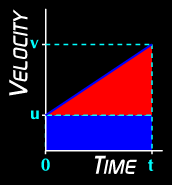# v = u + at

The equation: a = (v-u) ÷ t can be rearranged to give another useful equation: v = u + at

With this we can work out the velocity objects will have if we know the acceleration, time and initial velocity. Neat!

However, you also know that the area under the line of a velocity-time graph is equivalent to the displacement. Let's look closer at this:The area under the line could be worked out from the triangle and the rectangle. Easy enough with numbers. However, you may have seen a quicker method before!Forces & Motion MenuGo to next page